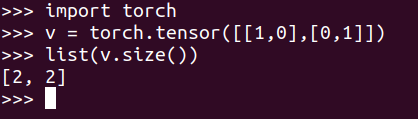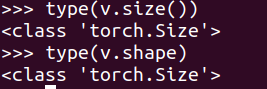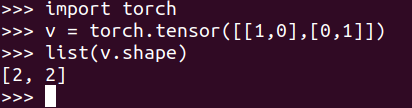How to Get the Shape of a Tensor as a List of int in Pytorch?

• Last Updated : 04 Jul, 2021

To get the shape of a tensor as a list in PyTorch, we can use two approaches. One using the size() method and another by using the shape attribute of a tensor in PyTorch. In this short article, we are going to see how to use both of the approaches.

Using size() method:

The size() method returns the size of the self tensor. The returned value is a subclass of a tuple.

Python3

 import torchtorch.empty(3, 4, 5).size()

Output:

torch.Size([3, 4, 5])

We cast this into a list using the list() method.

Example:

Python3

 v = torch.tensor([[1,0],[0,1]])x = list(v.size())print(x)

Output:

[2, 2]

You can also use the Python interactive session as shown below:Using shape attribute:

The tensor.shape is an alias to tensor.size(), though the shape is an attribute, and size() is a method. To verify this we can run the following in the interactive session.We can similarly cast this into a list using the list() method.

Example:

Python3

 import torchv = torch.tensor([[1,0],[0,1]])x = list(v.shape)print(x)

Output:

[2,2]

Alternatively using the interactive session:Attention geek! Strengthen your foundations with the Python Programming Foundation Course and learn the basics.

To begin with, your interview preparations Enhance your Data Structures concepts with the Python DS Course. And to begin with your Machine Learning Journey, join the Machine Learning - Basic Level Course

My Personal Notes arrow_drop_up